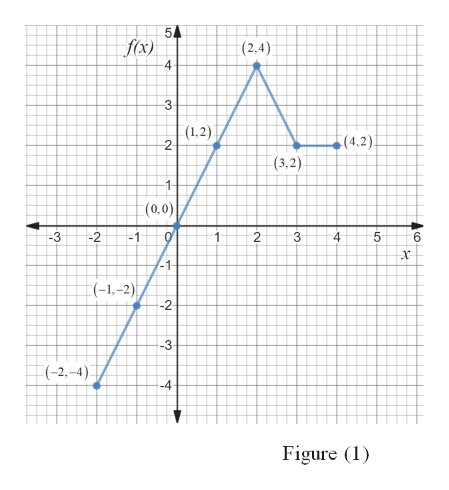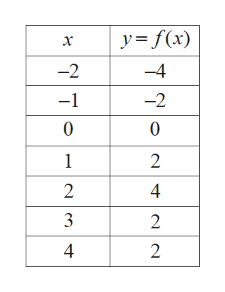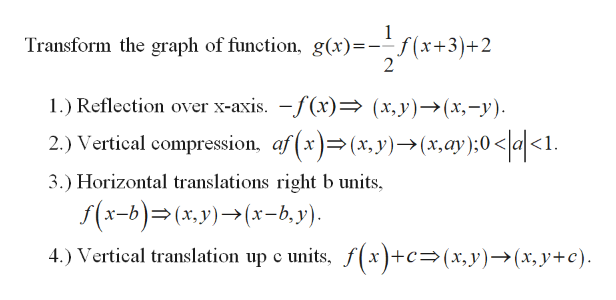# 12) g(x) = f(x-3)+ 2f(x - 3) + 2.. Graph: g(x) = -| f(-x)|

Question
53 views

Draw another line, point by point.
the graph of g(x), the transformed function whose parent function is y=f(x). The graph of y=f(x) is drawn in each of the coordinate systems on the picture graph.

check_circle

Step 1

Draw the transformed graph g(x), from the parent function of graph is given in Figure (1).help_outlineImage Transcriptionclose5 fx) (2,4) 4 3 (1,2) 2- (4,2). (3,2) (0,0) -3 -2 -1 3 4 6 1 (-1,-2) -2- -3 (-2,-4) -4- Figure (1) .co LO st co st fullscreen
Step 2

From the graph, the coordinate points is represented in table,help_outlineImage Transcriptionclosey f(x) -2 -4 -2 -1 0 0 1 2 2 4 2 4 2 N fullscreen
Step 3

The transformed function of g(...help_outlineImage Transcriptionclose1 Transform the graph of function, g(x)=--f(x+3)+2 1.) Reflection over x-axis. -f(x)- (x,y)->(x,-y) 2.) Vertical compression, af(x)=(x,y)->(x,ay );0 < a<1. 3.) Horizontal translations right b units f(x-b)(x,y)(x-b, y) 4.) Vertical translation up c units, f(x)+c>(x,y)-(x,y+c) fullscreen

### Want to see the full answer?

See Solution

#### Want to see this answer and more?

Solutions are written by subject experts who are available 24/7. Questions are typically answered within 1 hour.*

See Solution
*Response times may vary by subject and question.
Tagged in

### Functions